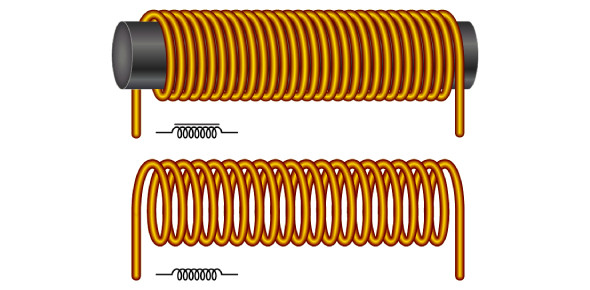# Inductive Reactance Quiz By Gibilisco

18 Questions | Attempts: 601
ShareSettingsIf you want to enhance your Inductive Reactance knowledge and wish to practice questions, take this informative quiz covering questions by Gibilisco. The quiz covers the topic thoroughly and is aimed at providing you with the best set of questions that will not only test you but will also provide you with valuable information that would be of great help during the exam. Take this quiz to know more. If you like the quiz, share it with your friends and family. All the best!

• 1.
As the number of turns in a coil that carries ac increases without limit, the current in the coil will
• A.

Eventually become very large

• B.

Stay the same

• C.

Decrease, approaching zero

• D.

Be stored in the core material

• 2.
As the number of turns in a coil increases, the reactance at a constant frequency
• A.

Increases

• B.

Decreases

• C.

Stays the same

• D.

Is stored in the core material

• 3.
As the frequency of an ac wave gets lower, the value of XL for a particular coil of wire
• A.

Increases

• B.

Decreases

• C.

Stays the same

• D.

Depends on the voltage

• 4.
Suppose a coil has an inductance of 100 mH. What is the reactance at a frequency of 1000Hz?
• A.

0.628 Ω

• B.

6.28 Ω

• C.

62.8 Ω

• D.

628 Ω

• 5.
Suppose a coil shows an inductive reactance of 200 Ω at 500 Hz. What is its inductance?
• A.

0.637 H

• B.

628 H

• C.

63.7 mH

• D.

628 mH

• 6.
Imagine a 400-µH inductor with a reactance of 33 Ω. What is the frequency?
• A.

13 kHz

• B.

0.013 kHz

• C.

83 kHz

• D.

83 MHz

• 7.
Suppose an inductor has XL = 555 Ω at f = 132 kHz. What is L?
• A.

670 mH

• B.

670 µH

• C.

460 mH

• D.

460 µH

• 8.
Suppose a coil has L = 689 µH at f = 990 kHz. What is XL?
• A.

682 Ω

• B.

4.28 Ω

• C.

4.28 kΩ

• D.

4.28 MΩ

• 9.
Suppose an inductor has L = 88 mH with XL = 100 Ω. What is f?
• A.

55.3 kHz

• B.

55.3 Hz

• C.

181 kHz

• D.

181 Hz

• 10.
Each point in the RL plane
• A.

Corresponds to a unique resistance

• B.

Corresponds to a unique inductance

• C.

Corresponds to a unique combination of resistance and inductive reactance

• D.

Corresponds to a unique combination of resistance and inductance

• 11.
If the resistance R and the inductive reactance XL both are allowed to vary from zero tounlimited values, but are always in the ratio 3:1, the points in the RL plane for all the resultingimpedances will lie along
• A.

A vector pointing straight up

• B.

A vector pointing east

• C.

A circle

• D.

A ray of indefinite length, pointing outward from the origin

• 12.
Each specific complex impedance value defined in the form R + jXL
• A.

Corresponds to a specific point in the RL plane

• B.

Corresponds to a specific inductive reactance

• C.

Corresponds to a specific resistance

• D.

All of the above are true

• 13.
A vector is defined as a mathematical quantity that has
• A.

Magnitude and direction

• B.

Resistance and inductance

• C.

Resistance and reactance

• D.

Inductance and reactance

• 14.
In an RL circuit, as the ratio of inductive reactance to resistance (XL/R) decreases, the phaseangle
• A.

Increases

• B.

Decreases

• C.

Stays the same

• D.

Becomes alternately positive and negative

• 15.
In a circuit containing inductive reactance but no resistance, the phase angle is
• A.

Constantly increasing

• B.

Constantly decreasing

• C.

Equal to 0°

• D.

Equal to 90°

• 16.
If the inductive reactance and the resistance in an RL circuit are equal (as expressed in ohms),then what is the phase angle?
• A.

• B.

45°

• C.

90°

• D.

It depends on the actual values of the resistance and the inductive reactance

• 17.
Consider an RL circuit that consists of a 100-µH inductor and a 100-Ω resistor. What is thephase angle at a frequency of 200 kHz?
• A.

45.0°

• B.

51.5°

• C.

38.5°

• D.

There isn’t enough data given to calculate it

• 18.
Suppose an RL circuit has an inductance of 88 mH, and the resistance is 95 Ω. At 800 Hz,what is the phase angle?
• A.

78°

• B.

12°

• C.

43°

• D.

47°

## Related TopicsBack to top
×

Wait!
Here's an interesting quiz for you.